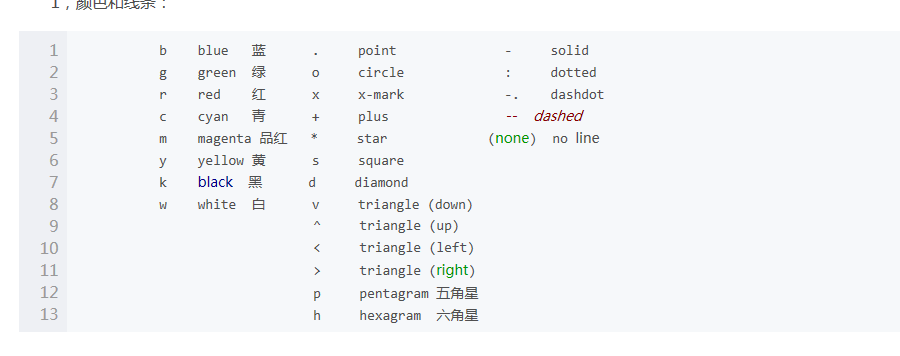• 指定颜色、线型与宽度、横纵坐标数值与标签大小 clo={‘k-’,‘k:’,‘k-.’,‘k–’,‘k-’};...plot(h1(i).h,c,‘LineWidth’, str2num(lin(i))); hold on; end title(‘EWHM,z=0.8’,‘Font...
指定颜色、线型与宽度、横纵坐标数值与标签大小
clo={‘k-’,‘k:’,‘k-.’,‘k–’,‘k-’};
lin=[‘2’,‘2’,‘2’,‘2’,‘5’];
c=[];
for i=1:1:5
c=char(clo(i));
plot(h1(i).h,c,‘LineWidth’, str2num(lin(i)));
hold on;
end
title(‘EWHM,z=0.8’,‘FontSize’,10);
xlabel(‘时间s’,‘FontSize’,10);
ylabel(‘失效率λ(t)’,‘FontSize’,10);
hl=legend(‘ξ=0,η=0,ν=0’,‘ξ=10,η=0,ν=0’,‘ξ=10,η=0.001,ν=0’,‘ξ=0,η=0,ν=2’,‘ξ=10,η=0.001,ν=2’);
% set(hl,‘NumColumns’,2);
set(hl,‘Box’,‘off’);
ylim([-5,30]);
set(gca,‘fontsize’,10);
scatter(x,y,100,55,‘filled’);
text(x,y,{id},‘HorizontalAlignment’,‘right’,‘fontsize’,16);https://blog.csdn.net/xiaotao_1/article/details/79100163


展开全文• ## Matlabplot

千次阅读 2010-05-21 14:43:00
二维曲线绘图语法plot(Y)plot(X1,Y1,...)plot(X1,Y1,LineSpec,...)plot(...,PropertyName,PropertyValue,...)plot(axes_handle,...)h = plot(...)hlines = plot(v6,...)描述plot(Y)如果Y是m×n的数组，以1:m为X横...

二维曲线绘图

语法
plot(Y)plot(X1,Y1,...)plot(X1,Y1,LineSpec,...)plot(...,'PropertyName',PropertyValue,...)plot(axes_handle,...)h = plot(...)hlines = plot('v6',...)

描述
plot(Y)如果Y是m×n的数组，以1:m为X横坐标，Y中的每一列元素为Y坐标，绘制n条曲线；如果Y是n×1或者1×n的向量，则以1:n为横坐标，Y为坐标表绘制1条曲线；如果Y是复数，则plot(Y)等效于plot(real(Y),imag(Y))；其它使用情况下，忽略坐标数据中的虚部。

plot(X1,Y1,...)如果X和Y都是数组，按列取坐标数据绘图，此时它们必须具有相同的尺寸；如果X和Y其中一个是向量另一个为数组，X和Y中尺寸相等的方向对应绘制多条曲线；如果X和Y其中一个是标量另一个为向量，那么将绘制垂直X或者Y轴离散的点。

X

Y

备注

m×n

m×n

按列取坐标数据绘制n条曲线

X和Y必须具有相同的尺寸

1×n或n×1

m×n或n×m

自动匹配尺寸相同方向绘制m条曲线

任意四种组合，效果一样

m×n或n×m

1×n或n×1

同上

同上

1×1

1×n或n×1

绘制垂直X轴离散点

Y可以任意向量

1×n或n×1

1×1

绘制垂直Y轴离散点

X可以任意向量

plot(X1,Y1,LineSpec,...)通过参数LineSpec指定曲线的曲线属性，它包括线型、标记符和颜色。plot函数支持同时绘制任意组图形

plot(X1,Y1,LineSpec1,X2,Y2,LineSpec2,...)

此时完全等效于

plot(X1,Y1,LineSpec1,...)
hlod all
plot(X2,Y2,LineSpec2,...)

MATLAB中提供的线型属性有：

线型

说明

标记符

说明

颜色

说明

-

实线(默认)

+

加号符

r

红色

--

双划线

o

空心圆

g

绿色

:

虚线

*

星号

b

蓝色

:.

点划线

.

实心圆

c

青绿色

x

叉号符

m

洋红色

s

正方形

y

黄色

d

菱形

k

黑色

^

上三角形

w

白色

v

下三角形

>

右三角形

<

左三角形

p

五角星

h

六边形

需要说明的是，LineSpec中设置曲线线型、标识符和颜色三项属性时，控制符的顺序不受限制并可以省略或者部分省略。也就是说'r-.*'、'-.r*'、'*-.r'等形式是等效的，都表示使用红色点划线连接各个节点，各节点使用“*”标识。

plot(...,'PropertyName',PropertyValue,...)设置由plot创建的所有曲线句柄对象的属性，Line对象属性和属性值参见附录，具体设置参考下面的实例，当然可以使用set/get进行设置。

plot(axes_handle,...)指定坐标系，也就是在axes_handle坐标系中绘图，在没有指定时默认为gca。

h = plot(...)返回由plot创建的所有曲线句柄对象的句柄。每条曲线对应一个句柄，如果有n条曲线，则h为n×1的数组。

注意
在同时绘制多条曲线时，如果没有指定曲线属性，plot按顺序循环使用当前坐标系中ColorOrder和LineStyleOrder两个属性。

默认情况，MATLAB在每次调用plot函数时将ColorOrder和LineStyleOrder自动重置为DefaultAxesColorOrder和DefaultAxesLineStyleOrder。Default**属性我们可以自定义，有效期至MATLAB关闭，Matlab下次启动时将Default**属性重置为厂家设置(Factory)

set(0,'DefaultAxesColorOrder',’r|g|b|k’,...
'DefaultAxesLineStyleOrder','-|-.|--|:')

使用hold all命令可以阻止调用plot函数时自动重置ColorOrder和LineStyleOrder属性，而是循环使用。注意hold on只是使多次绘制的图形叠加（相当于NextPlot），但不能阻止属性重置。

另外我们可以通过下面四个属性设置标识符的颜色和大小
LineWidth——指定线宽
MarkerEdgeColor——指定标识符的边缘颜色
MarkerFaceColor——指定标识符填充颜色
MarkerSize——指定标识符的大小
注意上面四个属性是针对当前坐标系中所有曲线的

实例
% by dynamic
% 2009.8.20
%
X=1:10;
% 两个都是数组，必须具有相同的尺寸
X1=[X;X;X]';%10×3
Y1=rand(10,3)+1;%10×3
% 其中一个为向量，另一个为数组，自动匹配尺寸相等方向
X2=1:0.1:10;%1×91
Y2=[sin(X2);cos(X2)]';%91×2
% 其中一个是标量，另一为矢量，绘制垂直坐标轴的离散点
X3=1:10;
Y3=-0.5;
fh=figure('numbertitle','off','name','PLOT Usability Demo');%创建figure对象
ah=axes;%创建axes对象
h=plot(...%返回所有曲线句柄
ah,...%指定坐标系，可以省略，此时默认gca
X1,Y1,...%坐标数据
'-.^',...%曲线属性，可以省略或部分省略，此时自动选择
X2,Y2,...
'm-',...
X3,Y3,...
'o',...%注意此组数据设置线型和颜色无效，因为默认绘制离散点
'LineWidth',2,...%线宽
'MarkerEdgeColor','k',...%标识符边缘颜色
'MarkerFaceColor','r',...%标识符填充颜色
'MarkerSize',8)%标识符大小

set(gca,'XTick',-pi:pi/2:pi) set(gca,'XTickLabel',{'-pi','-pi/2','0','pi/2','pi'})

展开全文c
• MATLAB Plot 如何自定义颜色Tip: Matlab 作图时最好保存成fig格式，便于以后修改。写论文是一个漫长的过程，成文后最重要的一个问题就是如何让自己的论文排版和插图更加的好看，让枯燥的论文有一点生动的色彩。这里...
MATLAB Plot 如何自定义颜色Tip: Matlab 作图时最好保存成fig格式，便于以后修改。写论文是一个漫长的过程，成文后最重要的一个问题就是如何让自己的论文排版和插图更加的好看，让枯燥的论文有一点生动的色彩。这里记录我在写论文时的困惑和一些小小的技巧。以下面这个图为例：X = linspace(0,5,51);Y1 = sin(X);Y2 = cos(0.5*X);Z = 2*Y1+0.3*Y2;plot(X,Y1,'color',[1,0,1],'Marker','*');hold on;plot(X,Y2,'color',[0.9,0.5,0.5],'LineWidth',2);hold on;plot(X,Z,'color',[0.9,1,0.7],'Marker','diamond','MarkerEdgeColor','r')xlabel('x','FontSize',15)ylabel('y','FontSize',15)title('Customized the plot color in Matlab','Color',[0.7,0,1])方括号中间的三个数字表示的三原色的比例，配色标准可以参考：Matlab配色Plot的基本命令： plot(x,y,'property name',property value)如果不记得property name怎么办？不用担心，Matlab会给你提示，总是可以找到自己想要的。如何画出渐变图？当然是写个for循环就可以了。如下图：clear allhold offX = linspace(-pi/2,pi/2,10)figure('Color',[0.94,1,0.94])for i = 1:20plot(X,(-1)^(i)*i*X,'color',[1-i*0.05,0+i*0.03,1-i*0.01],'LineWidth',0.5+0.05*i);hold on;endaxis([-pi/2,pi/2,-35,35])
展开全文• plot函数可以接一些参数，来改变所画图像的属性（颜色，图像元素等）。下面是一些属性的说明 b blue（蓝色） . point（点） - solid（实线） g green（绿色） o circle（圆圈） : dotted（点线)


plot函数可以接一些参数，来改变所画图像的属性（颜色，图像元素等）。下面是一些属性的说明            b     blue（蓝色）       .     point（点）       -     solid（实线）           g     green（绿色）      o     circle（圆圈）    :     dotted（点线)           r     red（红色）        x     x-mark（叉号）    -.    dashdot (点画线）           c     cyan（墨绿色）     +     plus(加号）       --    dashed(虚线）            m     magenta（紫红色） *     star（星号）      (none) no line           y     yellow（黄色）     s     square（正方形）           k     black（黑色）      d     diamond（菱形）                               v     triangle (down)                               ^     triangle (up)                               <     triangle (left)                               >     triangle (right)                               p     pentagram                               h     hexagram

Example       x = -pi:pi/10:pi;       y = tan(sin(x)) - sin(tan(x));       plot(x,y,'--rs','LineWidth',2,...                       'MarkerEdgeColor','k',...                       'MarkerFaceColor','g',...                       'MarkerSize',10)
·         用Matlab画图时，有时候需要对各种图标进行标注，例如，用“+”代表A的运动情况，“*”代表B的运动情况。
legend函数的基本用法是
LEGEND(string1,string2,string3, ...)
分别将字符串1、字符串2、字符串3……标注到图中，每个字符串对应的图标为画图时的图标。
例如:
plot(x,sin(x),'.b',x,cos(x),'+r')
legend('sin','cos')这样可以把"."标识为'sin'，把"+"标识为"cos"
还可以用LEGEND(...,'Location',LOC) 来指定图例标识框的位置
这些是Matlab help文件。后面一段是对应的翻译和说明
'North'              inside plot box near top        'South'              inside bottom        'East'               inside right        'West'               inside left        'NorthEast'          inside top right (default)        'NorthWest           inside top left        'SouthEast'          inside bottom right        'SouthWest'          inside bottom left        'NorthOutside'       outside plot box near top        'SouthOutside'       outside bottom        'EastOutside'        outside right        'WestOutside'        outside left        'NorthEastOutside'   outside top right        'NorthWestOutside'   outside top left        'SouthEastOutside'   outside bottom right        'SouthWestOutside'   outside bottom left        'Best'               least conflict with data in plot        'BestOutside'        least unused space outside plot
'North'             图例标识放在图顶端        'South'            图例标识放在图底端        'East'               图例标识放在图右方        'West'              图例标识放在图左方        'NorthEast'       图例标识放在图右上方（默认）        'NorthWest      图例标识放在图左上方        'SouthEast'      图例标识放在图右下角        'SouthWest'     图例标识放在图左下角(以上几个都是将图例标识放在框图内）        'NorthOutside'          图例标识放在图框外侧上方        'SouthOutside'         图例标识放在图框外侧下方        'EastOutside'           图例标识放在图框外侧右方        'WestOutside'          图例标识放在图框外侧左方        'NorthEastOutside'   图例标识放在图框外侧右上方        'NorthWestOutside' 图例标识放在图框外侧左上方        'SouthEastOutside'   图例标识放在图框外侧右下方        'SouthWestOutside' 图例标识放在图框外侧左下方（以上几个将图例标识放在框图外）        'Best'                      图标标识放在图框内不与图冲突的最佳位置        'BestOutside'           图标标识放在图框外使用最小空间的最佳位置 还是用上面的例子
legend('sin','cos','location','northwest')可以将标识框放置在图的左上角。

Examples:        x = 0:.2:12;        plot(x,bessel(1,x),x,bessel(2,x),x,bessel(3,x));        legend('First','Second','Third');        legend('First','Second','Third','Location','NorthEastOutside')        b = bar(rand(10,5),'stacked'); colormap(summer); hold on        x = plot(1:10,5*rand(10,1),'marker','square','markersize',12,...                 'markeredgecolor','y','markerfacecolor',[.6 0 .6],...                 'linestyle','-','color','r','linewidth',2); hold off        legend([b,x],'Carrots','Peas','Peppers','Green Beans',...                  'Cucumbers','Eggplant')


展开全文conflict string
• 转自：https://blog.csdn.net/jirryzhang/article/details/77374702 MATLAB plot画线的颜色设定 plot中画线的颜色通常是八种： 标记符 颜色 r 红 g 绿 b 蓝 c 蓝绿 m 紫红 y ...
• ## matlabplot函数详解

万次阅读 多人点赞 2018-04-28 17:04:08
matlab中，plot函数用来绘制二维图像。1.plot默认格式 plot(x,y)这种格式中，若x,y是向量，则它们必须具有相同的长度。函数将以x为横轴，绘制y。 若x,y都是矩阵，则它们必须具有相同的尺寸，plot函数将针对x的各...
• matlabplot学习笔记 点击 https://www.matplotlib.org.cn/tutorials/#%E5%BA%8F%E8%A8%80
• 文章目录Matlab plot画图相关函数基本用法添加轴坐标和标题坐标轴在图形添加文本附注进阶绘制多个数据集图例图窗窗口在一个图窗中显示多个绘图绘制虚数和复数数据附表 Matlab plot画图相关函数 将matlab官方文档对...
• 一、Matlab绘图中用到的直线属性包括： （1）LineStyle：线形 （2）LineWidth：线宽 （3）Color：颜色 （4）MarkerType：标记点的形状 （5）MarkerSize：标记点的大小 （6）MarkerFaceColor：标记...
• matlab plot命令：形如plot（x,y1,'p')；
• % plot([A(1,1) B(1,1)] , [ A(1,2) B(1,2) ] ); % plot([A(2,1) B(2,1)] , [ A(2,2) B(2,2) ] ); % plot([A(3,1) B(3,1)] , [ A(3,2) B(3,2) ] ); plot([A(:,1) B(:,1)]', [A(:,2) B(:,2)]'); % camproj('...
• MATLAB有非常强的图形功能，能够方便地实现数据的...5.1 二维图形的绘制5.1.1 基本形式二维图形的绘制是MATLAB语言图形处理的基础，MATLAB最经常使用的画二维图形的命令是plot，看两个简单的样例：>> y=[0 0.5...
• https://ww2.mathworks.cn/products/matlab/plot-gallery.html
• MATLAB plot画线的颜色设定 plot中画线的颜色通常是八种： 标记符 颜色 r 红 g 绿 b 蓝 c 蓝绿 m 紫红 y 黄 k 黑 w 白 但是线的颜色是由红绿蓝（RGB）三原色组成的，通过设定三原色的权重可以改变线的颜色，命令...
• 另一种方法是使用内置的网格：h=gca;grid on % turn on major grid linesgrid minor % turn on minor grid lines% Set limits and grid spacing separately for the two directions:h.XAxis.Limits=[-1,1];...
• matlab中二维线画图函数 1. plot(x,y); %x y 为相应点集 2.plot(x,y1,x,y2); % 在一个窗口下绘制多条曲线之方法一 3.hold on %在一个窗口下绘制多条曲线之方法二 plot(x,y1); plot(x,y2); hold off 4.plot...
• virtulenv 建立后显示matlabplot 需要是framework 解决方法： import matplotlib matplotlib.use('TkAgg') import matplotlib.pyplot as plt 转载于:https://www.cnblogs.com/litingyu/p/9780990.html...
• ## matlabplot一点小细节

千次阅读 2016-02-25 11:36:33
由于粗心，没有看matlab plot的资料，把 plot(x,y,..)中 x 和 y 的顺序弄反了，调试了一段时间才发现这个问题，现记录一下，防止再犯。  在 Matlab 中，plot( x, y , ...)中，x 是横坐标，也就是矩阵中列号，y是纵...
• 使用matlab画图过程中的一些问题记录 文章目录1. 线形、标记和颜色代码2. 坐标轴、图名和网格线如需加粗字体如需要换行：如设置字号：3. 一图多线（1）hold on语句（2）text()语句（3）gtext()语句4. 更改坐标图...
• MATLAB plot用指定的8种字符可以使得曲线呈现8种不同的颜色，这8种系统颜色分别是 颜色 字符 红 r 绿 g 蓝 b 青绿 c 洋红 m 黄 y 黑 k 白 w 例如命令 plot(x, y, '......

# matlabplotmatlab 订阅